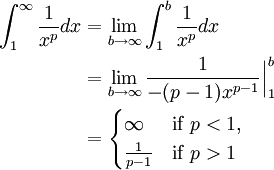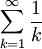# The p-series

Previous: Example: Integral Test with a Logarithm

Next: Videos on the Integral Test

## Problem

For what values of p does the infinite seriesconverge?

## Complete Solution

### Step (1): Consider p > 0 and p ≠ 1

When p > 0 and p ≠ 1, the functionis continuous, decreasing, and positive when x is in the interval [1,∞). Using the integral test,Therefore, the infinite series converges when p > 1, and diverges when p is in the interval (0,1).

### Step (2): Consider p ≤ 0 and p = 1

If p=1, then we have the harmonic serieswhich we know diverges.

If p ≤ 0, the infinite series diverges (by the divergence test).

Therefore, the given series only converges for p > 1.

## The p-Series

The result of this example can be summarized as follows.

The p-Series

The p-seriesis convergent if p > 1 and divergent if p ≤ 1.

Much like a geometric series, we can use this result to determine whether a given infinite series converges by inspection. For example, the infinite seriesdiverges because it is a p-series with p equal to 1/2 (you may want to let u=(1+k) to see this).

Previous: Example: Integral Test with a Logarithm

Next: Videos on the Integral Test